Updating search results...

# 11 Results

View
Selected filters:
• algebra-1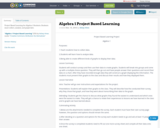Only Sharing Permitted
CC BY-ND
Rating
0.0 stars

Project Based Learning for Algebra 1 Students. Students must collect, analyze, and graph date.

Subject:
Mathematics
Material Type:
Homework/Assignment
11/15/2016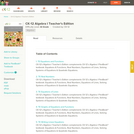Conditional Remix & Share Permitted
CC BY-NC-SA
Rating
0.0 stars

CK-12 Foundation's Algebra I Teacher's Edition FlexBook complements CK-12's Algebra I Student Edition. The solution and assessment guides are available upon request.

Subject:
Algebra
Mathematics
Material Type:
Textbook
Provider:
CK-12 Foundation
Provider Set:
CK-12 FlexBook
Author:
Fay-Zenk, Mary
McFarland, Andrew
11/06/2009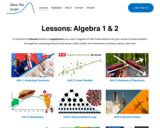Conditional Remix & Share Permitted
CC BY-NC-SA
Rating
0.0 stars

A collection of relevant lessons to supplement your units in Algebra I/II. Mix these lessons into your course to show students the algebraic reasoning behind social issues, public health, the environment, business, sports, and more.

Subject:
Mathematics
Material Type:
Activity/Lab
Lesson
Lesson Plan
Author:
Skew The Script
01/31/2023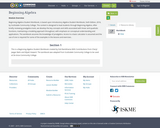Conditional Remix & Share Permitted
CC BY-NC
Rating
0.0 stars

Beginning Algebra Student Workbook, is based upon Introductory Algebra Student Workbook, Sixth Edition, 2016, by Scottsdale Community College. The content is designed to lead students through Beginning Algebra. After briefly reviewing prealgebra tools, we develop the key concepts and skills associated with linear and quadratic functions, maintaining a modeling approach throughout, with emphasis on conceptual understanding and applications. The workbook assumes the knowledge of prealgebra. Access to a basic calculator is assumed and the use of one is required for some of the examples in the lessons and exercises .

Subject:
Algebra
Material Type:
Module
Author:
lenore desilets
07/11/2019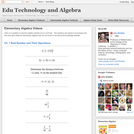Conditional Remix & Share Permitted
CC BY-NC
Rating
0.0 stars

Visually searchable database of Algebra 1 videos. Click on a problem and watch the solution on YouTube. Copy and paste this material into your CMS. Videos accompany the open Elementary Algebra textbook published by Flat World Knowledge.

Subject:
Algebra
Mathematics
Material Type:
Lecture
Lecture Notes
Provider:
Individual Authors
Provider Set:
Individual Authors
Author:
John Redden
04/29/2012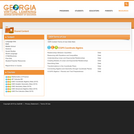Conditional Remix & Share Permitted
CC BY-NC-SA
Rating
0.0 stars

Full course of Algebra 1 is presented online by Georgia Virtual Learning. Audio, video, text, games and activities are included to engage ninth grade students in learning.

Subject:
Algebra
Functions
Mathematics
Material Type:
Activity/Lab
Full Course
Provider:
Georgia Department of Education
Provider Set:
Georgia Virtual Learning
08/02/2013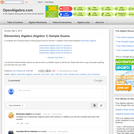Conditional Remix & Share Permitted
CC BY-NC-SA
Rating
0.0 stars

Subject:
Algebra
Mathematics
Material Type:
Assessment
Homework/Assignment
Lecture Notes
Provider:
openAlgebra.com
Provider Set:
Individual Authors
Author:
John Redden
05/05/2013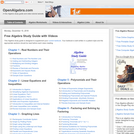Conditional Remix & Share Permitted
CC BY-NC-SA
Rating
0.0 stars

This free study guide is designed to supplement your current textbook. This Algebra Study Guide is a solutions oriented approach to Algebra. When solving problems students are asked to communicate their thought process by showing steps wherever possible.

Subject:
Algebra
Mathematics
Material Type:
Diagram/Illustration
Homework/Assignment
Lecture
Lecture Notes
Provider:
openAlgebra.com
Provider Set:
Individual Authors
Author:
John Reden
02/02/2013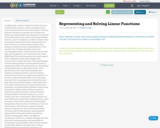Only Sharing Permitted
CC BY-NC-ND
Rating
0.0 stars

In eighth grade, students studied how linear functions represented by equations, tables, and graphs can be used to model and solve real world situations. Particular attention was given to using the rate of change and initial value from multiple representations to model the relationship between two values with the generalizable function, y=mx+b. In Algebra 1, students continue using and making connections between representations of linear functions. A common misconception when making connections between representations is that constant rate of change and slope can be used interchangeably when “a linear function does not have slope, but the graph of a non-vertical line has a slope.” (High School Functions progression document, page 6) Unit 2 establishes a deep understanding of the characteristics of linear functions. This understanding includes exploring linear functions geometrically by analyzing the effects of transformations on the graph by replacing f(x) by f(x) + k, kf(x), f(kx) and f(x + k) for specific values of k. These understandings allow students to compare linearity to function families studied in future units (e.g., exponential, quadratic).

In this unit, students will extend their focus from slope intercept form to reasoning about standard form and point slope form. Students begin to make distinctions about which of these forms are most beneficial when modeling a real world situation. Different contexts lend themselves to different forms of linear equations. Students may build a function to model a situation, using parameters from that situation (e.g., rate of change, start value, ordered pair). Other situations are more efficiently modeled with standard form (e.g., Dana purchased 3 brauts and 4 drinks for \$8.50). Symbolic manipulation from one form to another can reveal new characteristics of the function or assist in solving systems of equations.

In 6th and 7th grade students solved one and two step equations and inequalities algebraically. In 8th grade students solved linear equations using graphs, tables, and algebraic manipulation. In this unit, students will apply what they know about solving equations and inequalities to solving multi-step inequalities which include variables on both sides. Students will make sense of what a solution means for an equation compared to an inequality. Students will extend their understanding of solving linear equations with two variables. First, they will manipulate equations to solve for specific variables. Second, students will justify their reasoning by supplying mathematical properties to explain each step in solving an equation. This work will help set the groundwork for mathematical proofs in tenth grade.

In addition, students will extend their 8th grade understanding of solving systems of linear equations to include systems of linear inequalities as representations of real world situations. Students will solve systems of linear equations exactly (e.g. with substitution principle, combination/elimination), and approximately (e.g., with graphs) with a new emphasis on the conceptual understanding and justification of why these strategies work. Students will compare and contrast the benefit of using each of these strategies in different situations.

In this unit students are using what they know about linear functions to build new understandings of piecewise linear functions including absolute value functions. In addition, students will use tables and graphs to solve absolute value equations as described in HSA.REI.D.11. The Michigan State Standards no longer require students to be able to algebraically solve piecewise and absolute value functions.

Subject:
Mathematics
Material Type:
Module
Unit of Study
06/20/2017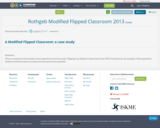Only Sharing Permitted
CC BY-NC-ND
Rating
0.0 stars

This is a summary and example of my experiences and successes "flipping" my Algebra I classroom in the 2012-13 school year. An example of the materials a student would encounter in a given 24 hour period are included.

Subject:
Mathematics
Material Type:
Case Study
Teaching/Learning Strategy
01/28/2016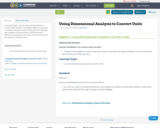Conditional Remix & Share Permitted
CC BY-NC
Rating
0.0 stars

Learning Target: I can use dimensional analysis to convert units.

Students will explore different strategies to calculate feet per second to miles per hour.

Students will complete a group activity to find the speed of Barbie's car and convert the units of measure from feet per second to miles per hour.

Subject:
Mathematics
Material Type:
Activity/Lab